# Check if Cell Contains Specific Number – Excel & Google Sheets

This tutorial demonstrates how to check if a cell contains a specific number using the FIND and ISNUMBER Functions in Excel and Google Sheets.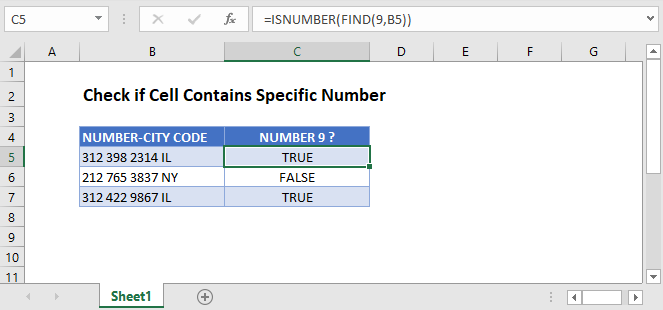## Check if Cell Contains Specific Number Using ISNUMBER and FIND

A cell that contains a mix of letters, numbers, spaces, and/or symbols is stored as a text cell in Excel. We can check if one of those cells contains a specific number with the ISNUMBER and FIND Functions.

``=ISNUMBER(FIND(9,B3))``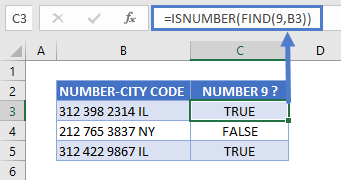Let’s step through this two-part formula.

### The FIND Function

First, the FIND Function checks for a specified character within a text string. It then returns the position of that character in the string, or a #VALUE! error if it is not found.

``=FIND(9,B3)``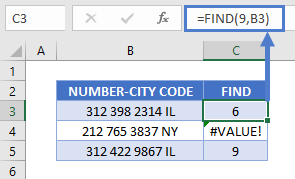In this example, the FIND Function returns the position of “9” in each cell. As shown above, for cells without the number 9, it returns a VALUE error.

Note: You can use either the FIND or SEARCH Function to check if a cell contains a specific number. Both functions have the same syntax and, with numeric find texts, produce the same result.

### The ISNUMBER Function

Next, the ISNUMBER Function does what its name implies: it checks if a cell is a number and returns TRUE or FALSE.

``=ISNUMBER(C3)``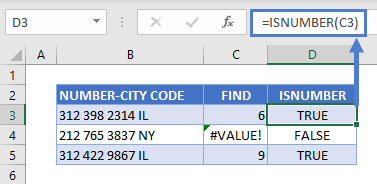The ISNUMBER Function checks the result of the FIND Function and returns TRUE for cells with position numbers and FALSE for the cell with a VALUE error.

Combining these functions together gives us our original formula:

``=ISNUMBER(FIND(9,B3))``## Check if Cell Contains Specific Number in Google Sheets

These functions work the same in Google Sheets as in Excel.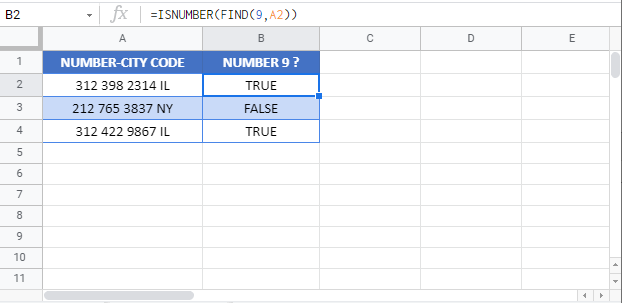### Excel Practice Worksheet

Practice Excel functions and formulas with our 100% free practice worksheets!